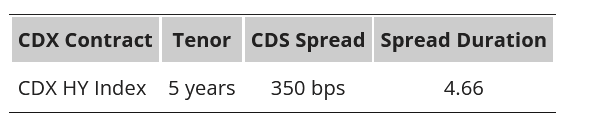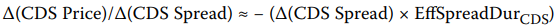# CDS price and price change

CDS Price and Price ChangesKim buys \$10,000,000 notional value of protection using the CDX contract. Kim’s view turns out to be correct and after 12 months the CDS spread on the HY CDX contract has doubled and the contract’s spread duration is 3.77.
The fund is buying protection and therefore needs to pay the fixed coupon of 0.05 × \$10,000,000 = \$500,000.
|Initial CDS Price|= 1 + [(Fixed coupon – CDS spread) × Spread duration]||= 1 + [(0.05 – 0.035) × 4.66]||= 1.0699|

The CDS price after 12 months when the CDS spread has doubled to 700 bps and the spread duration has fallen to 3.77 is calculated as:
|CDS price in 12 months|= 1 + [(0.05 – 0.07) × 3.77]|||= 0.9246|
Because the fund has bought protection, it is a short credit risk, and profits as CDS prices fall. Hence, the profit/loss from changes in the CDS price = (1.0699 – 0.9246) × \$10,000,000 = \$1,453,000.
Total return, including the coupon outflow = \$1,453,000 − \$500,000 = \$953,000.

Why does the formulalead to a different result?
In this case, the change would be: 350bps x 3,77 x notional = 1319500, not 1453000…

You can’t use that formula because spread duration has changed since inception. You have to calculate price is \$ terms and then calculate P&L

Correct… I realized, too. Thanks for posting!